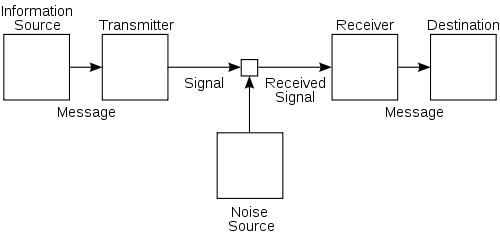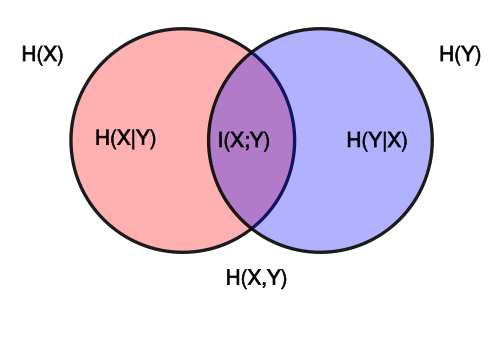## Overview

Information theory is based on probability and statistics.

Major application/goal:

• coding theory (theory of coding process)
• source coding, or data compression
• channel coding, or error correction

## A Communication System

A communication system: (symbolically represented as)

1. As objects: message - signal - (received) signal - message
2. As processes: coding process - noise - decoding process
3. As agent: information source - transmitter - channel - receiver - destination

Note: A coding process is the action of the transmitter in changing the message into the signal.Figure: Shannon's diagram of a general communications system

Questions concerning this communication system:

1. How does one measure the amount of information? (Information)
2. How does one measure the capacity of a communication channel? (Capacity of a communication channel)
3. What are the characteristics of an efficient coding process? And when the coding is as efficient as possible, at what rate can the channel convey information? (Coding)
4. What are the general characteristics of noise? How does noise affect the accuracy of the message finally received at the destination? How can one minimize the undesirable effects of noise, and to what extent can they be eliminated? (Noise)
5. If the signal being transmitted is continuous rather than being formed of discrete symbols, how does the fact affect the problem? (Continuous messages)

### Information

Information is a measure of one’s freedom of choice when one selects a message. If one is confronted with a very elementary situation where he has to choose one of two alternative messages, then it is arbitrarily said that the information, associated with this situation, is unity.

The real reason that Level A (technical problem) analysis deals with a concept of information which characterizes the whole statistical nature of the information source, and is not concerned with the individual messages (and not at all directly concerned with the meaning of the individual messages) is that from the point of view of engineering, a communication system must face the problem of handling any message that the source can produce. … This sort of consideration leads at once to the necessity of characterizing the statistical nature of the whole ensemble of messages which a given kind of source can and will produce.

The ratio of the actual to the maximum entropy is called the relative entropy of the source.

One minus the relative entropy is called the redundancy. This is the fraction of the structure of the message which is determined not by the free choice of the sender, but rather by the accepted statistical rules governing the use of the symbols in question. [Just like the grammar to a language.]

The concept of information developed in this theory at first seems disappointing and bizarre -- disappointing because it has nothing to do with meaning, and bizarre because it deals not with a single message but rather with the statistical character of a whole ensemble of messages, bizarre also because in these statistical terms the two words information and uncertainty find themselves to be partners.

### Capacity of a Communication Channel

The capacity of a channel is to be described in terms of its ability to transmit what is produced out of source of a given information.

### Coding

For a noiseless channel transmitting discrete symbols, the capacity of the communication channel is C bit/s, the source of entropy (or information) produces signals H bit/s, then by devising proper coding procedures for the transmitter it is possible to transmit symbols over the channel at an average rate which is nearly $\frac{C}{H}$, but which can never be made to exceed $\frac{C}{H}$.

The best transmitter, in fact, is that which codes the message in such a way that the signal has just those optimum statistical characteristics which are best suited to the channel to be used -- which in fact maximize the signal entropy and make it equal to the capacity C of the channel.

### Noise

It is generally true that when there is noise, the received signal exhibits greater information -- or better, the received signal is selected out of a more varied set than is the transmitted signal.

Uncertainty which arises by virtue of freedom of choice on the part of the sender is desirable uncertainty. Uncertainty which arises because of errors or because of the influence of noise is undesirable uncertainty.

Equivocation is the entropy of the message relative to the signal, which measures the average uncertainty in the message when the signal is known.

The capacity of a noisy channel is defined to be the maximum rate (in bits per second) at which useful information (total uncertainty minus noise uncertainty) can be transmitted over the channel.

Suppose that a noisy channel has a capacity C, suppose it is accepting from an information source characterized by an entropy of $H(x)$ bit/s, the entropy of the received signals being $H(y)$ bit/s. Then,

• If the channel capacity C is equal to or larger than $H(x)$, then by devising appropriate coding systems, the output of the source can be transmitted over the channel with as little error as one pleases. However small a frequency of error you specify, there is a code which meets the demand.
• If the channel capacity C is less than $H(x)$, it is impossible to devise codes which reduce the error frequency as low as one may please. The undesirable uncertainty, or equivocation, will always be equal to or greater than $H(x) - C$. Furthermore, there is always at least one code which is capable of reducing this undesirable uncertainty, concerning the message, down to a value which exceeds $H(x) - C$ by an arbitrarily small amount.

### Continuous Messages

For a channel of frequency bandwidth W, when the average power used in transmitting is P, the channel being subject to a noise of power N, this noise being “white thermal noise” of a special kind which Shannon defines. Then it is possible, by the best coding, to transmit binary digits at the rate of (maximum capacity) $$C = W \log \frac{P+N}{N}$$ and have an arbitrarily low frequency of error.

## Quantities of information

The most important ones:

• entropy - information in a random variable
• mutual information - amount of information in common between two random variables

### Axioms for the uncertainty measure $H(X)$

1. $f(M) = H(1/M, …, 1/M)$ should be a monotonically increasing function of M
2. $f(ML) = f(M) + f(L)$
3. (Grouping axiom) $$H(p_1, …, p_M) = H( \sum_{i=1}^r p_i , \sum_{j=r+1}^M p_j) + \sum_{i=1}^r p_i * H( \frac{p_1}{\sum_{i=1}^r p_i}, \dots, \frac{p_r}{\sum_{i=1}^r p_i} ) + \sum_{j=r+1}^M p_j * H(\frac{p_{r+1}}{\sum_{j=r+1}^M p_j}, \dots, \frac{p_M}{\sum_{j=r+1}^M p_j})$$
1. In r.v. form: $$H(X) = H(G) + p_a * H(X|G=a) + p_b * H(X|G=b) = H(G) + E_G{H(X|g)}$$
2. In general: $$H(X) = H(Y) + H(X|Y)$$
4. $H(p, 1-p)$ is a continuous function of p.

Note on each axioms:

1. [等概率随机事件的uncertainty measure随可能事件数的增加单调增加。]
2. [独立等概率事件的联合uncertainty measure等于各uncertainty measure之和。]
3. [The average uncertainty about the compound experiment minus the average uncertainty removed by specifying the group equals the average uncertainty remaining after the group is specified.]
4. [This is for mathematical convenience.]

### Definition

Entropy of a discrete random variable X is the expectation of its self-information $I(X)$.

$$H(X) = \operatorname{E}_X [I(X)] = \operatorname{E}_X [-\log_2 P(X)]$$

Self-information $I(X)$ is a random variable that indicates the entropy contribution of an individual message X.

$$I(X) = -\log_2 P(X)$$

The unit of information is bit, while 'nat' and 'hartley' may also be used in non-standard definitions.

Note:

1. This definition of $H(X)$ satisfies all the four axioms mentioned above, and it can be proved to be the only one.
2. [interpretation] H(X) is the minimum average number of “yes or no” questions required to determine the result of one observation of X.
3. Entropy is maximized when all the messages in the message space are equiprobable.

Joint entropy of two discrete r.v.’s X and Y is the entropy of r.v. $(X, Y)$.

$$H(X,Y) = \operatorname{E}_{X,Y} [-\log_2 P(X,Y)]$$

It can be shown that $H(X,Y) \leq H(X) + H(Y)$ with equality if and only if X and Y are independent.

Conditional entropy of r.v. X given r.v. Y is the average conditional entropy of X given Y.

$$H(X|Y) = \operatorname{E}_Y [H(X|Y=y)]$$

It can be shown that $$H(X|Y) = H(X,Y) - H(Y)$$

Mutual information $I(X;Y)$ of X relative to Y is the amount of information that can be obtained about one random variable by observing another, in other words, the information conveyed about X by Y.

$$I(X;Y) = H(X) - H(X|Y)$$

Note:

1. Mutual information is symmetric. $$I(X;Y) = I(Y;X) = I(X) + I(Y) - I(X,Y)$$
2. Mutual information is non-negative, and is equal to zero if and only if X and Y are independent.Figure: Individual, joint, conditional and mutual entropies for a pair of correlated subsystems X,Y

## Coding Theory

Source coding is the process of taking the source data and making it smaller. While the idea of Channel coding is to find codes that transmit quickly, contain many valid code words and can correct or at least detect many errors. (noise & redundancy)

Information rate is the average entropy per symbol.

Channel capacity is $$C = \sup_{P_X} I(X;Y)$$

### The fundamental theorem for a noiseless channel

Let a source have entropy H (bits per symbol) and a channel have a capacity C (bits per second). Then it is possible to encode the output of the source in such a way as to transmit at the average rate $\frac{C}{H} - e$ symbols per second over the channel where e is arbitrarily small. It is not possible to transmit at an average rate greater than $\frac{C}{H}$.

Note: The essential content of this theorem is that the average number of “yes or no” questions needed to specify the value of X can never be less than the uncertainty (measure) of X.

### The fundamental theorem for a discrete channel with noise

Let a discrete channel have the capacity C and a discrete source the entropy per second H. If $H \leq C$ there exists a coding system such that the output of the source can be transmitted over the channel with an arbitrarily small frequency of errors (or an arbitrarily small equivocation). If $H>C$ it is possible to encode the source so that the equivocation is less than $H-C+e$ where e is arbitrarily small. There is no method of encoding which gives an equivocation less than $H-C$.

## Relationship between entropies in thermodynamics and information theory

In the discrete case using base two logarithms, the reduced Gibbs entropy is equal to the minimum number of yes/no questions needed to be answered in order to fully specify the microstate, given that we know the macrostate.

In fact one can generalise: any information that has a physical representation must somehow be embedded in the statistical mechanical degrees of freedom of a physical system.

When information is physical, all processing of its representations, i.e. generation, encoding, transmission, decoding and interpretation, are natural processes where entropy increases by consumption of free energy.